1. /
2. CBSE
3. /
4. Class 07
5. /
6. Mathematics
7. /
8. NCERT Solutions for Class...

# NCERT Solutions for Class 7 Maths Exercise 11.3### myCBSEguide App

Download the app to get CBSE Sample Papers 2023-24, NCERT Solutions (Revised), Most Important Questions, Previous Year Question Bank, Mock Tests, and Detailed Notes.

NCERT solutions for Maths Perimeter and Area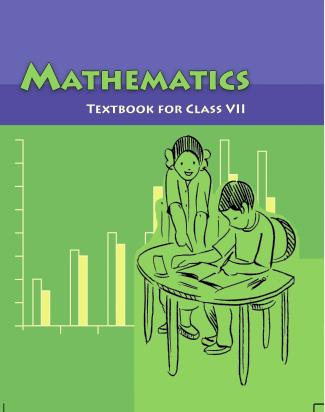## NCERT Solutions for Class 7 Maths Perimeter and Area

###### Question 1.Find the circumference of the circles with the following radius: {tex}\left( {{\text{Take }}\pi {\text{ = }}\frac{{22}}{7}} \right){/tex}

(a) 14 cm

(b) 28 mm

(c) 21 cm

(a) Circumference of the circle = {tex}2\pi r{/tex} = {tex}2 \times \frac{{22}}{7} \times 14{/tex} = 88 cm

(b) Circumference of the circle = {tex}2\pi r{/tex} = {tex}2 \times \frac{{22}}{7} \times 28{/tex} = 176 mm

(c) Circumference of the circle = {tex}2\pi r{/tex} = {tex}2 \times \frac{{22}}{7} \times 21{/tex} = 132 cm

NCERT Solutions for Class 7 Maths Exercise 11.3

###### Question 2.Find the area of the following circles, given that: {tex}\left( {{\text{Take }}\pi {\text{ = }}\frac{{22}}{7}} \right){/tex}

(b) diameter = 49 m

(a) Area of circle = {tex}\pi {r^2}{/tex} = {tex}\frac{{22}}{7} \times 14 \times 14{/tex} = 22 x 2 x 14 = 616 {tex}m{m^2}{/tex}

(b) Diameter = 49 m

{tex}\therefore {/tex} radius = {tex}\frac{{49}}{2}{/tex} = 24.5 m

{tex}\therefore {/tex} Area of circle = {tex}\pi {r^2}{/tex} = {tex}\frac{{22}}{7} \times 24.5 \times 24.5{/tex} = 22 x 3.5 x 24.5 = 1886.5 {tex}{m^2}{/tex}

Area of circle = {tex}\pi {r^2}{/tex} = {tex}\frac{{22}}{7} \times 5 \times 5{/tex} = = {tex}\frac{{550}}{7}{/tex} {tex}c{m^2}{/tex}

NCERT Solutions for Class 7 Maths Exercise 11.3

###### Question 3.If the circumference of a circular sheet is 154 m, find its radius. Also find the area of the sheet. {tex}\left( {{\text{Take }}\pi {\text{ = }}\frac{{22}}{7}} \right){/tex}

Circumference of the circular sheet = 154 m

{tex} \Rightarrow {/tex} {tex}2\pi r{/tex} = 154 m {tex} \Rightarrow {/tex} {tex}r = \frac{{154}}{{2\pi }}{/tex}

{tex} \Rightarrow {/tex} {tex}r = \frac{{154 \times 7}}{{2 \times 22}}{/tex} = 24.5 m

Now Area of circular sheet = {tex}\pi {r^2}{/tex} = {tex}\frac{{22}}{7} \times 24.5 \times 24.5{/tex}

= = 22 x 3.5 x 24.5 = 1886.5 {tex}{m^2}{/tex}

Thus, the radius and area of circular sheet are 24.5 m and 1886.5 {tex}{m^2}{/tex}respectively.

NCERT Solutions for Class 7 Maths Exercise 11.3

###### Question 4.A gardener wants to fence a circular garden of diameter 21 m. Find the length of the rope he needs to purchase, if he makes 2 rounds of fence. Also, find the costs of the rope, if it cost Rs. 4 per meter. {tex}\left( {{\text{Take }}\pi {\text{ = }}\frac{{22}}{7}} \right){/tex}

Diameter of the circular garden = 21 m

{tex}\therefore {/tex} Radius of the circular garden = {tex}\frac{{21}}{2}{/tex} m

Now Circumference of circular garden = {tex}2\pi r{/tex} = {tex}2 \times \frac{{22}}{7} \times \frac{{21}}{2}{/tex}

= 22 x 3 = 66 m

The gardener makes 2 rounds of fence so the total length of the rope of fencing

= 2 x {tex}2\pi r{/tex} = 2 x 66 = 132 m

Since the cost of 1 meter rope = Rs. 4

Therefore, cost of 132 meter rope = 4 c 132 = Rs. 528

NCERT Solutions for Class 7 Maths Exercise 11.3

###### Question 5.From a circular sheet of radius 4 cm, a circle of radius 3 cm is removed. Find the area of the remaining sheet. {tex}\left( {{\text{Take }}\pi {\text{ = 3}}{\text{.14}}} \right){/tex}

Radius of circular sheet (R) = 4 cm and radius of removed circle {tex}\left( r \right){/tex} = 3 cm

Area of remaining sheet = Area of circular sheet – Area of removed circle

= {tex}\pi {{\text{R}}^2} – \pi {r^2}{/tex} = {tex}\pi \left( {{{\text{R}}^2} – {r^2}} \right){/tex}

= {tex}\pi \left( {{4^2} – {3^2}} \right){/tex} = {tex}\pi \left( {16 – 9} \right){/tex}

= 3.14 x 7 = 21.98{tex}\;c{m^2}{/tex}

Thus, the area of remaining sheet is 21.98{tex}\;c{m^2}{/tex}.

NCERT Solutions for Class 7 Maths Exercise 11.3

###### Question 6.Saima wants to put a lace on the edge of a circular table cover of diameter 1.5 m. Find the length of the lace required and also find its cost if one meter of the lace costs Rs. 15. {tex}\left( {{\text{Take }}\pi {\text{ = 3}}{\text{.14}}} \right){/tex}

Diameter of the circular table cover = 1.5 m

{tex}\therefore {/tex} Radius of the circular table cover = {tex}\frac{{1.5}}{2}{/tex} m

Circumference of circular table cover = {tex}2\pi r{/tex} = {tex}2 \times 3.14 \times \frac{{1.5}}{2}{/tex} = 4.71 m

Therefore the length of required lace is 4.71 m.

Now the cost of 1 m lace = Rs. 15

Then the cost of 4.71 m lace = 15 x 4.71 = Rs. 70.65

Hence, the cost of 4.71 m lace is Rs. 70.65.

NCERT Solutions for Class 7 Maths Exercise 11.3

###### Question 7.Find the perimeter of the adjoining figure, which is a semicircle including its diameter.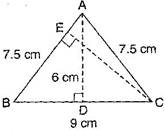Diameter = 10 cm

{tex}\therefore {/tex} Radius = {tex}\frac{{10}}{2}{/tex} = 5 cm

According to question,

Perimeter of figure = Circumference of semi-circle + diameter

= {tex}\pi r{/tex} + D

= {tex}\frac{{22}}{7} \times 5 + 10{/tex} = {tex}\frac{{110}}{7} + 10{/tex}

= {tex}\frac{{110 + 70}}{7} = \frac{{180}}{7}{/tex} = 25.71 cm

Thus, the perimeter of the given figure is 25.71 cm.

NCERT Solutions for Class 7 Maths Exercise 11.3

###### Question 8.Find the cost of polishing a circular table-top of diameter 1.6 m, if the rate of polishing is Rs. 15/{tex}{m^2}{/tex}. {tex}\left( {{\text{Take }}\pi {\text{ = 3}}{\text{.14}}} \right){/tex}

Diameter of the circular table top = 1.6 m

{tex}\therefore {/tex} Radius of the circular table top = {tex}\frac{{1.6}}{2} = {/tex} 0.8 m

Area of circular table top = {tex}\pi {r^2}{/tex}

= 3.14 x 0.8 x 0.8 = 2.0096 {tex}{m^2}{/tex}

Now cost of 1 m2 polishing = Rs. 15

Then cost of 2.0096{tex}{m^2}{/tex} polishing = 15 x 2.0096 = Rs. 30.14 (approx.)

Thus, the cost of polishing a circular table top is Rs. 30.14 (approx.)

NCERT Solutions for Class 7 Maths Exercise 11.3

###### Question 9.Shazli took a wire of length 44 cm and bent it into the shape of a circle. Find the radius of that circle. Also find its area. If the same wire is bent into the shape of a square, what will be the length of each of its sides? Which figure encloses more area, the circle or the square? {tex}\left( {{\text{Take }}\pi {\text{ = }}\frac{{22}}{7}} \right){/tex}

Total length of the wire = 44 cm

{tex}\therefore {/tex} the circumference of the circle = {tex}2\pi r{/tex} = 44 cm

{tex} \Rightarrow {/tex} {tex}2 \times \frac{{22}}{7} \times r = 44{/tex} {tex} \Rightarrow {/tex} {tex}r = \frac{{44 \times 7}}{{2 \times 22}}{/tex} = 7 cm

Now Area of the circle = {tex}\pi {r^2}{/tex} = {tex}\frac{{22}}{7} \times 7 \times 7{/tex} = 154 cm2

Now the wire is converted into square.

Then perimeter of square = 44 cm

{tex} \Rightarrow {/tex} 4 x side = 44 {tex} \Rightarrow {/tex} side = {tex}\frac{{44}}{4}{/tex} = 11 cm

Now area of square = side x side = 11 x 11 = 121 {tex}c{m^2}{/tex}

Therefore, on comparing, the area of circle is greater than that of square, so the circle enclosed more area.

Question 10.From a circular card sheet of radius 14 cm, two circles of radius 3.5 cm and a rectangle of length 3 cm and breadth 1 cm are removed (as shown in the adjoining figure). Find the area of the remaining sheet. {tex}\left( {{\text{Take }}\pi {\text{ = }}\frac{{22}}{7}} \right){/tex}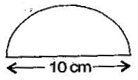Radius of circular sheet (R) = 14 cm and Radius of smaller circle {tex}\left( r \right){/tex} = 3.5 cm

Length of rectangle {tex}\left( l \right){/tex} = 3 cm and breadth of rectangle {tex}\left( b \right){/tex} = 1 cm

According to question,

Area of remaining sheet=Area of circular sheet– (Area of two smaller circle + Area of rectangle)

= {tex}\pi {{\text{R}}^2} – \left[ {2\left( {\pi {r^2}} \right) + \left( {l \times b} \right)} \right]{/tex}

= {tex}\frac{{22}}{7} \times 14 \times 14 – \left[ {\left( {2 \times \frac{{22}}{7} \times 3.5 \times 3.5} \right) – \left( {3 \times 1} \right)} \right]{/tex}

= 22 x 14 x 2 – [44 x 0.5 x 3.5 + 3]

= 616 – 80

= 536 {tex}c{m^2}{/tex}

Therefore the area of remaining sheet is 536 cm2.

NCERT Solutions for Class 7 Maths Exercise 11.3

###### Question 11.A circle of radius 2 cm is cut out from a square piece of an aluminium sheet of side 6 cm. What is the area of the left over aluminium sheet? {tex}\left( {{\text{Take }}\pi {\text{ = 3}}{\text{.14}}} \right){/tex}

Radius of circle = 2 cm and side of aluminium square sheet = 6 cm

According to question,

Area of aluminium sheet left = Total area of aluminium sheet – Area of circle

= side x side – {tex}\pi {r^2}{/tex}

= 6 x 6 – {tex}\frac{{22}}{7}{/tex} x 2 x 2

= 36 – 12.56

= 23.44 {tex}c{m^2}{/tex}

Therefore, the area of aluminium sheet left is 23.44 {tex}c{m^2}{/tex}.

NCERT Solutions for Class 7 Maths Exercise 11.3

###### Question 12.The circumference of a circle is 31.4 cm. Find the radius and the area of the circle. {tex}\left( {{\text{Take }}\pi {\text{ = 3}}{\text{.14}}} \right){/tex}

The circumference of the circle = 31.4 cm

{tex} \Rightarrow {/tex} {tex}2\pi r{/tex} = 31.4 {tex} \Rightarrow {/tex} 2 x 3.14 x {tex}r{/tex} = 31.4

{tex} \Rightarrow {/tex} {tex}r = \frac{{31.4}}{{2 \times 3.14}}{/tex} = 5 cm

Then area of the circle = {tex}\pi {r^2}{/tex} = 3.14 x 5 x 5

= 78.5 {tex}c{m^2}{/tex}

Therefore, the radius and the area of the circle are 5 cm and 78.5{tex}c{m^2}{/tex} respectively.

NCERT Solutions for Class 7 Maths Exercise 11.3

###### Question 13.A circular flower bed is surrounded by a path 4 m wide. The diameter of the flower bed is 66 m. What is the area of this path? {tex}\left( {{\text{Take }}\pi {\text{ = 3}}{\text{.14}}} \right){/tex}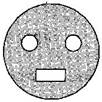Diameter of the circular flower bed = 66 m

{tex}\therefore {/tex} Radius of circular flower bed {tex}\left( r \right) = \frac{{66}}{2}{/tex} = 33 m

{tex}\therefore {/tex} Radius of circular flower bed with 4 m wide path (R) = 33 + 4 = 37 m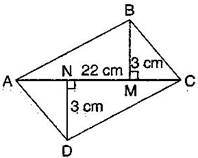According to the question,

Area of path = Area of bigger circle – Area of smaller circle

= {tex}\pi {{\text{R}}^2} – \pi {r^2}{/tex} = {tex}\pi \left( {{{\text{R}}^2} – {r^2}} \right){/tex}

= {tex}\pi \left[ {{{\left( {37} \right)}^2} – {{\left( {33} \right)}^2}} \right]{/tex}

= 3.14 [ (37 + 33) (37 – 33)] {tex}\left[ {\because {a^2} – {b^2} = \left( {a + b} \right)\left( {a – b} \right)} \right]{/tex}

= 3.14 x 70 x 4

= 879.20 {tex}{m^2}{/tex}

Therefore, the area of the path is 879.20 {tex}{m^2}{/tex}.

NCERT Solutions for Class 7 Maths Exercise 11.3

###### Question 14.A circular flower garden has an area of 314 m2. A sprinkler at the centre of the garden can cover an area that has a radius of 12 m. Will the sprinkler water the entire garden? {tex}\left( {{\text{Take }}\pi {\text{ = 3}}{\text{.14}}} \right){/tex}

Circular area by the sprinkler = {tex}\pi {r^2}{/tex} = 3.14 x 12 x 12

= 3.14 x 144 = 452.16 {tex}{m^2}{/tex}

Area of the circular flower garden = 314 {tex}{m^2}{/tex}

Since Area of circular flower garden is smaller than area by sprinkler.

Therefore the sprinkler will water the entire garden.

NCERT Solutions for Class 7 Maths Exercise 11.3

###### Question 15.Find the circumference of the inner and the outer circles, shown in the adjoining figure. {tex}\left( {{\text{Take }}\pi {\text{ = 3}}{\text{.14}}} \right){/tex}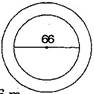Radius of outer circle {tex}\left( r \right){/tex} = 19 m

{tex}\therefore {/tex} Circumference of outer circle = {tex}2\pi r{/tex} = 2 x 3.14 x 19

= 119.32 m

Now radius of inner circle {tex}\left( {r’} \right){/tex} = 19 – 10 = 9 m

{tex}\therefore {/tex} Circumference of inner circle = {tex}2\pi r'{/tex} = 2 x 3.14 x 9

= 56.52 m

Therefore the circumferences of inner and outer circles are 56.52 m and 119.32 m respectively.

NCERT Solutions for Class 7 Maths Exercise 11.3

###### Question 16.How many times a wheel of radius 28 cm must rotate to go 352 m? {tex}\left( {{\text{Take }}\pi {\text{ = }}\frac{{22}}{7}} \right){/tex}

Let wheel must be rotate {tex}n{/tex} times of its circumference.

Radius of wheel = 28 cm and Total distance = 352 m = 35200 cm

{tex}\therefore {/tex} Distance covered by wheel = {tex}n{/tex} x circumference of wheel

{tex} \Rightarrow {/tex} 35200 = {tex}n \times 2\pi r{/tex}

{tex} \Rightarrow {/tex} 35200 = {tex}n \times 2 \times \frac{{22}}{7} \times 28{/tex}

{tex} \Rightarrow {/tex} {tex}n = \frac{{35200 \times 7}}{{2 \times 22 \times 28}}{/tex}

{tex} \Rightarrow {/tex} {tex}n{/tex} = 200 revolutions

Thus wheel must rotate 200 times to go 352 m.

NCERT Solutions for Class 7 Maths Exercise 11.3

###### Question 17.The minute hand of a circular clock is 15 cm long. How far does the tip of the minute hand move in 1 hour? {tex}\left( {{\text{Take }}\pi {\text{ = 3}}{\text{.14}}} \right){/tex}

In 1 hour, minute hand completes one round means makes a circle.

Radius of the circle {tex}\left( r \right){/tex} = 15 cm

Circumference of circular clock = {tex}2\pi r{/tex}

= 2 x 3.14 x 15 = 94.2 cm

Therefore, the tip of the minute hand moves 94.2 cm in 1 hour.

## NCERT Solutions for Class 7 Maths Exercise 11.3

NCERT Solutions Class 7 Maths PDF (Download) Free from myCBSEguide app and myCBSEguide website. Ncert solution class 7 Maths includes text book solutions from Class 7 Maths Book . NCERT Solutions for CBSE Class 7 Maths have total 15 chapters. 7 Maths NCERT Solutions in PDF for free Download on our website. Ncert Maths class 7 solutions PDF and Maths ncert class 7 PDF solutions with latest modifications and as per the latest CBSE syllabus are only available in myCBSEguide.

## CBSE app for Students

To download NCERT Solutions for Class 7 Maths, Social Science Computer Science, Home Science, Hindi English, Maths Science do check myCBSEguide app or website. myCBSEguide provides sample papers with solution, test papers for chapter-wise practice, NCERT solutions, NCERT Exemplar solutions, quick revision notes for ready reference, CBSE guess papers and CBSE important question papers. Sample Paper all are made available through the best app for CBSE students and myCBSEguide website.Test Generator

Create question paper PDF and online tests with your own name & logo in minutes.myCBSEguide

Question Bank, Mock Tests, Exam Papers, NCERT Solutions, Sample Papers, Notes

### 7 thoughts on “NCERT Solutions for Class 7 Maths Exercise 11.3”

1. Write clearly that people understand

2. it’s really good one you should try it…..
these all question are in my maths book all anawer are correct + solu is tooo… good……………..
love it….
10/10

3. this is very nice website

4. Thanks website